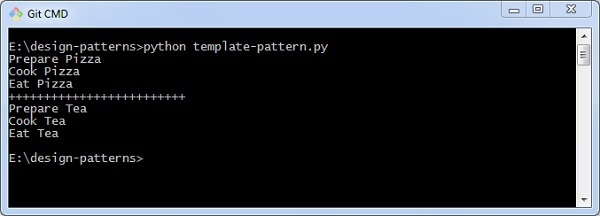# Python Design Patterns - Template

A template pattern defines a basic algorithm in a base class using abstract operation where subclasses override the concrete behavior. The template pattern keeps the outline of algorithm in a separate method. This method is referred as the template method.

Following are the different features of the template pattern −

• It defines the skeleton of algorithm in an operation

• It includes subclasses, which redefine certain steps of an algorithm.

```class MakeMeal:

def prepare(self): pass
def cook(self): pass
def eat(self): pass

def go(self):
self.prepare()
self.cook()
self.eat()

class MakePizza(MakeMeal):
def prepare(self):
print "Prepare Pizza"

def cook(self):
print "Cook Pizza"

def eat(self):
print "Eat Pizza"

class MakeTea(MakeMeal):
def prepare(self):
print "Prepare Tea"

def cook(self):
print "Cook Tea"

def eat(self):
print "Eat Tea"

makePizza = MakePizza()
makePizza.go()

print 25*"+"

makeTea = MakeTea()
makeTea.go()
```

### Output

The above program generates the following output −### Explanation

This code creates a template to prepare meal. Here, each parameter represents the attribute to create a part of meal like tea, pizza, etc.

The output represents the visualization of attributes.